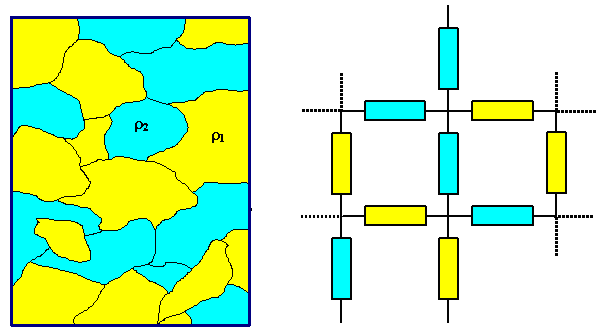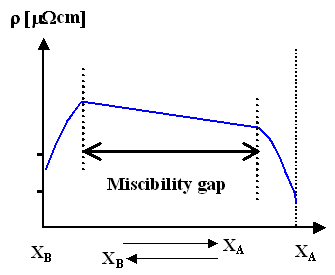# 2.2.2 Alloys

Pure metals are rarely used - in the real world you use alloys.

• In principle, the specific resistivity ρ of an alloy can be obtained from the phase diagram and the ρ - values of the phases involved. Lets look at the extremes:

1. Complete immiscibility, e.g. in the case of Au/Si, or Cu/W. We may treat the resulting mix of metal particles as a network of resistors being linked in series and parallel. The volume fractions of the phases would constitute the weights - the treatment is not unlike the elastic modulus of compounds.• But no matter what kind of volume fraction you use and how you treat the resistor network - the resulting resistivity willnever be smaller than that of the ingredient with the smallest resistivity.

2. Complete miscibility (e.g. Au/Ag, Cu/Ni). Experimentally we find for small amounts (some %) of B in A (with [B] = concentration of B)

$\rho\approx\rho_\text{A}+\text{const.}\cdot [B]$

• This formula is a special case of Nordheims rule which states .

$\rho\approx X_{\text{A}}\cdot\rho_{\text{A}}+ X_\text{B}\cdot\rho_\text{B}+ \text{const.} \cdot X_\text{A}\cdot X_\text{B}$

• This is pretty much an empirical law, it does not pay to justify it theoretically. Again, it is not possible to produce an alloy with a resistivity smaller than one of its components.

If you have intermetallic compounds in your phase diagram, use Nordheim's rule with the intermetallic phases as XA and XB.

• This leaves open the possibility that some intermetallic phase, i.e. a defined compound with its own crystal lattice, might have a lower resistivity than its constituents. While this is unlikely (if not outright impossible?) on theoretical grounds, no such intermetallics have been found so far.

The sad fact then is that unleashing the full power of metallurgy and chemistry on mixing conductors (i.e. metals), will not give you a conductor with a specific conductivity better than Ag.

• You will have to turn to superconductors (forgetting about cost considerations), if you can't live with Ag.

Lets look at some examples:

 Complete miscibility (e.g. Au/Ag) Miscibility gap (e.g. Ag/Cu); phase diagram in the link. Intermetallic phases (e.g. Cu/Au)What do we learn? Something simple:

• Again: The resistivity always goes up in alloys or mixed materials as compared to the pure materials.
• Things are generally complicated, but full of potential for custom tailored properties!